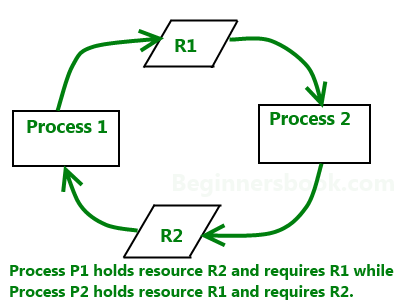Related Articles
• Difficulty Level : Medium
• Last Updated : 11 Dec, 2018

Livelock occurs when two or more processes continually repeat the same interaction in response to changes in the other processes without doing any useful work. These processes are not in the waiting state, and they are running concurrently. This is different from a deadlock because in a deadlock all processes are in the waiting state.Example:
Imagine a pair of processes using two resources, as shown:

 `void` `process_A(``void``)``{``    ``enter_reg(& resource_1);``    ``enter_reg(& resource_2);``    ``use_both_resources();``    ``leave_reg(& resource_2);``    ``leave_reg(& resource_1);``}``void` `process_B(``void``)``{``    ``enter_reg(& resource_1);``    ``enter_reg(& resource_2);``    ``use_both_resources();``    ``leave_reg(& resource_2);``    ``leave_reg(& resource_1);``}`

Each of the two processes needs the two resources and they use the polling primitive enter_reg to try to acquire the locks necessary for them. In case the attempt fails, the process just tries again.

If process A runs first and acquires resource 1 and then process B runs and acquires resource 2, no matter which one runs next, it will make no further progress, but neither of the two processes blocks. What actually happens is that it uses up its CPU quantum over and over again without any progress being made but also without any sort of blocking. Thus this situation is not that of a deadlock( as no process is being blocked) but we have something functionally equivalent to deadlock: LIVELOCK.

Occurrence of livelocks can occur in the most surprising of ways. The total number of allowed processes in some systems, is determined by the number of entries in the process table. Thus process table slots can be referred to as Finite Resources. If a fork fails because of the table being full, waiting a random time and trying again would be a reasonable approach for the program doing the fork.

Consider a UNIX system having 100 process slots. Ten programs are running, each of which having to create 12 (sub)processes. After each process has created 9 processes, the 10 original processes and the 90 new processes have exhausted the table. Each of the 10 original processes now sits in an endless loop forking and failing – which is aptly the situation of a deadlock. The probability of this happening is very little but it could happen.

Difference between Deadlock, Starvation, and Livelock:
A livelock is similar to a deadlock, except that the states of the processes involved in the livelock constantly change with regard to one another, none progressing. Livelock is a special case of resource starvation; the general definition only states that a specific process is not progressing.

Livelock:

 `var l1 = .... ``// lock object like semaphore or mutex etc``var l2 = .... ``// lock object like semaphore or mutex etc``     ` `        ``// Thread1``        ``Thread.Start( ()=> {``             ` `    ``while` `(``true``) {``         ` `        ``if` `(!l1.Lock(1000)) {``            ``continue``;``        ``}``         ` `        ``if` `(!l2.Lock(1000)) {``            ``continue``;``        ``}``         ` `        ``/// do some work``    ``});`` ` `        ``// Thread2``        ``Thread.Start( ()=> {``             ` `        ``while` `(``true``) {``             ` `            ``if` `(!l2.Lock(1000)) {``                ``continue``;``            ``}``             ` `            ``if` `(!l1.Lock(1000)) {``                ``continue``;``            ``}``             ` `            ``// do some work``        ``});`

 `var p = ``new` `object();``lock(p)``{``    ``lock(p)``    ``{``        ``// deadlock. Since p is previously locked``        ``// we will never reach here...``    ``}`
 `Queue q = .....`` ` `          ``while` `(q.Count & gt; 0)``{``    ``var c = q.Dequeue();``    ``.........`` ` `        ``// Some method in different thread accidentally``        ``// puts c back in queue twice within same time frame``        ``q.Enqueue(c);``    ``q.Enqueue(c);`` ` `    ``// leading to growth of queue twice then it``    ``// can consume, thus starving of computing``}`